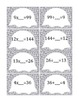# Ghosts Multiplication / Division Match Up: Aligned to the Common Core!Subject
Resource Type
File Type
PDF (4 MB|10 pages)
\$2.50
Product Description
Have your students practice finding the missing factors and identifying the related multiplication and division number sentences.

Students match the related multiplication and division number sentences, calculate the answer, then find the missing number.

Students can write the number sentences and missing numbers on the recording sheet provided or in their notebooks.

You can extend the activity by having the students write the other related multiplication/division sentences for each problem or draw arrays.

This game includes:
24 multiplication sentences
24 related division sentences
72 cards in total

CC.3.OA.5 Apply properties of operations as strategies to multiply and divide.

CC.3.OA.6 Understand division as an unknown-factor problem.

CC.3.OA.7 Fluently multiply and divide within 100, using strategies such as the relationship between multiplication and division.

CC.4.OA.4 Find all factor pairs for a whole number in the range 1-100.
Total Pages
10 pages